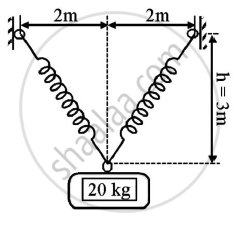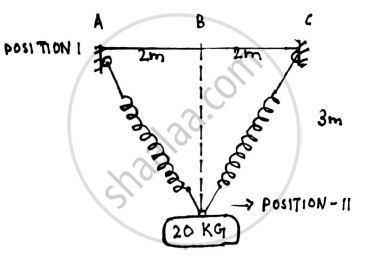# b)A cylinder has a mass of 20kg and is released from rest when h=0 as shown in the figure.Determine its speed when h=3m.The springs have an unstretched length of 2 m.Take k=40 N/m. - Engineering Mechanics

b)A cylinder has a mass of 20kg and is released from rest when h=0 as shown in the figure.Determine its speed when h=3m.The springs have an unstretched length of 2 m.Take k=40 N/m.Given : m=20 kg
h=0
k=40 N/m
To find : Speed when h=3m

#### SolutionPOSITION 1
Un-stretched length of spring = 2 m
Extension (x1) of spring = 0
Spring energy =ES1 =1/2 kx _1^2
=0
PE1=mgh
=0 J
KE1=0 J
AT POSITION II:

Let h=-3m
PE2 = mgh = 20 x 9.81 x (-3)
=-588.6 J
KE_2 = 1/2 xx 20v^2

=10v2

In△ABD,
By Pythagoras theorem
AD=sqrt(2^2+3^2)
=3.6056 m
Extension(x2) of spring = 3.6056 - 2=1.6056 m
E_(S2) = 1/2 Kx 2/2 = 1/2 xx 40 xx 1.6056^2
=51.5559 J
Applying work-energy principle
U_(1-2) = KE_2 - KE_1
PE_1 - PE_2 + E_(S1) - E_(S2) = KE_2 - KE_1
588.6 - 51.5559 = 10v2
v = 7.3283 m/s

Speed when h=3m is 7.3283 m/s

Concept: Principle of Work and Energy
Is there an error in this question or solution?
2016-2017 (December) CBGS

Share